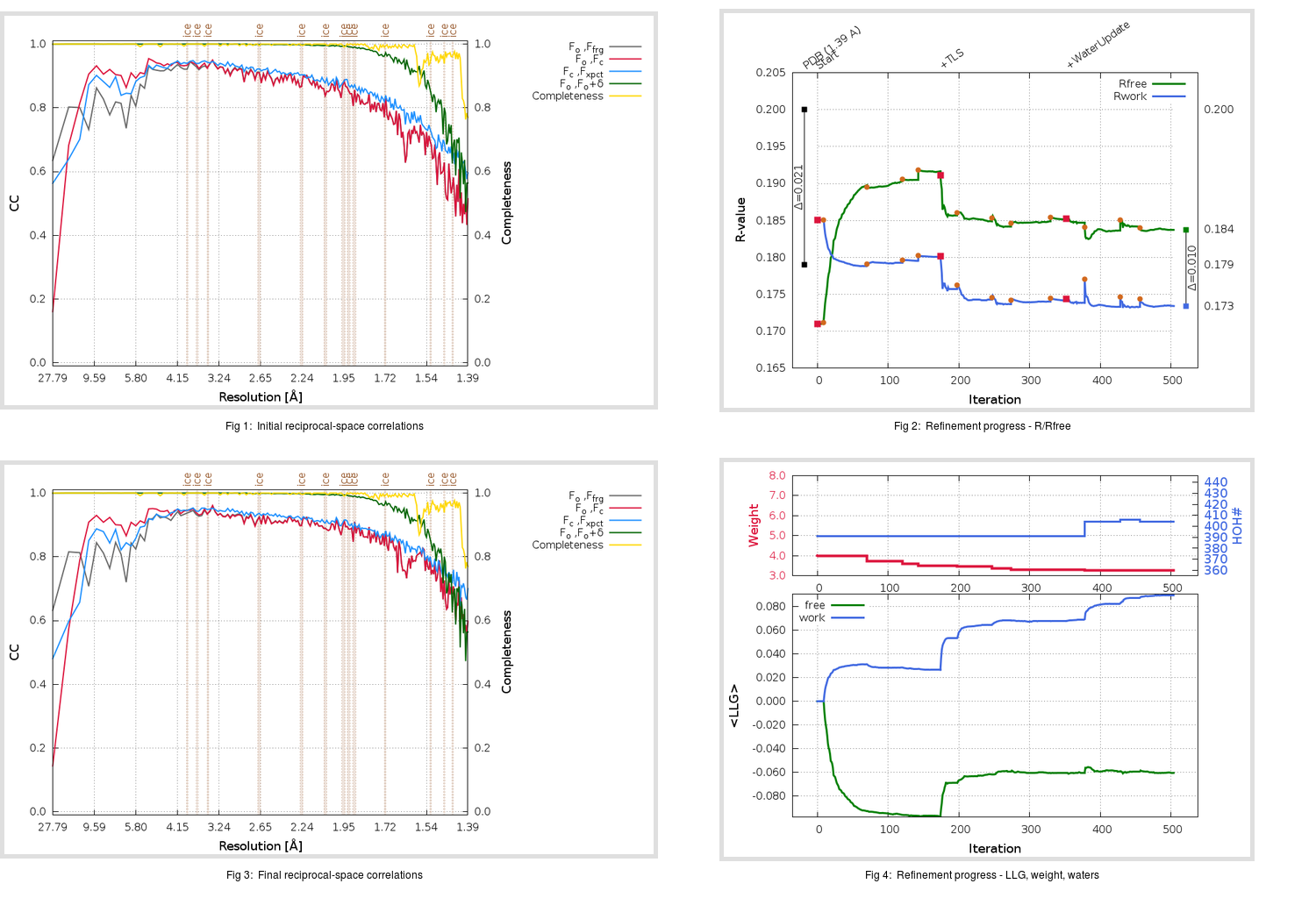Content:

```    Diffraction limits & principal axes of ellipsoid fitted to diffraction cut-off surface:
1.444         0.9631   0.0000  -0.2692       0.981 a* - 0.194 c*
1.387         0.0000   1.0000   0.0000       b*
1.352         0.2692   0.0000   0.9631       0.613 a* + 0.790 c*
```

## Deposited

` `
 Date deposited Date data collection Resolution R, Rfree 20200303 20200224 1.39 0.1780 0.2000

Molprobity (CCP4 7.0 version) summary:

```Ramachandran outliers =   0.33 %
favored =  98.34 %
Rotamer outliers      =   0.35 %
C-beta deviations     =     0
Clashscore            =   3.53
RMS(bonds)            =   0.0101
RMS(angles)           =   1.53
MolProbity score      =   1.14
Resolution            =   1.39
R-work                =   0.1780
R-free                =   0.2000
```

```Number of waters      =   391

<B> (all atoms) =   20.99 ( sd =    9.05 ) for       2950 non-hydrogen atoms
<B>   (protein) =   18.92 ( sd =    6.69 ) for       2543 non-hydrogen atoms
<B>     (water) =   32.89 ( sd =   10.43 ) for        391 non-hydrogen atoms
<B>    (others) =   33.83 ( sd =   10.35 ) for         16 non-hydrogen atoms

B min/max       (all non-hydrogen atoms) =   10.28 /   97.94
B min/max   (protein non-hydrogen atoms) =   10.28 /   56.09
B min/max     (water non-hydrogen atoms) =   11.47 /   97.94
B min/max     (other non-hydrogen atoms) =   19.79 /   48.75
```

## BUSTER (re-)refinement

` `

Molprobity (CCP4 7.0 version) summary:

```Ramachandran outliers =   0.33 %
favored =  99.01 %
Rotamer outliers      =   0.35 %
C-beta deviations     =     0
Clashscore            =   3.53
RMS(bonds)            =   0.0112
RMS(angles)           =   1.58
MolProbity score      =   1.14
Resolution            =   1.39
R-work                =   0.1735
R-free                =   0.1838
```

```Number of waters      =   404

<B> (all atoms) =   21.65 ( sd =    9.27 ) for       2963 non-hydrogen atoms
<B>   (protein) =   19.46 ( sd =    6.96 ) for       2543 non-hydrogen atoms
<B>     (water) =   33.87 ( sd =    9.78 ) for        404 non-hydrogen atoms
<B>    (others) =   35.27 ( sd =   11.66 ) for         16 non-hydrogen atoms

B min/max       (all non-hydrogen atoms) =   10.75 /   70.63
B min/max   (protein non-hydrogen atoms) =   10.75 /   58.71
B min/max     (water non-hydrogen atoms) =   12.70 /   70.63
B min/max     (other non-hydrogen atoms) =   19.93 /   52.86
```

Refinement progression:Results:

` `
 File Remark 6Y84_aB_refine.01_03_refine.pdb.gz exact refinement commands are in header 6Y84_aB_refine.01_03_refine.mtz.gz including original deposited data and several re-refinement map coefficients 6Y84_aB_refine.01_03_BUSTER_model.cif.gz including any non-standard compound restraints 6Y84_aB_refine.01_03_BUSTER_refln.cif.gz